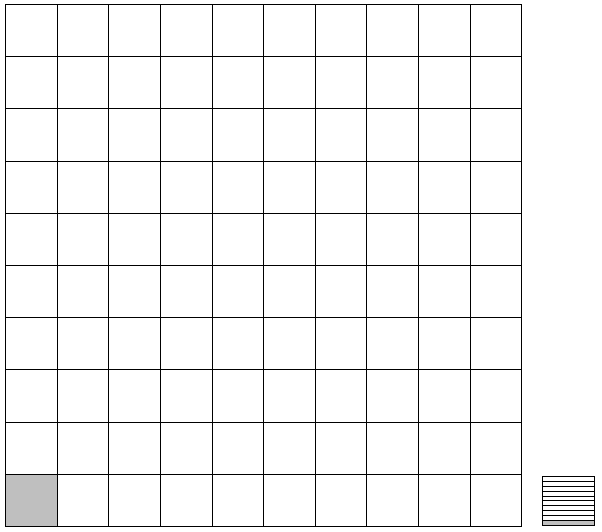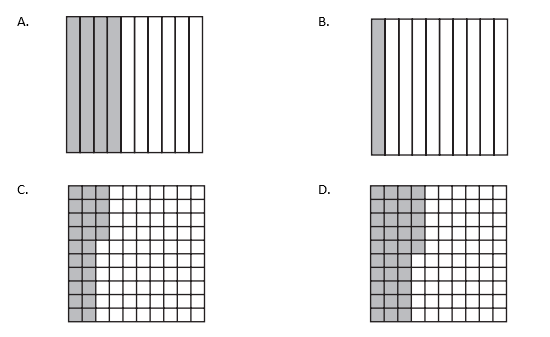# Decimal Fractions

## Objective

Compare two decimals less than 1, recording the result of a comparison with the symbol >, =, or <. Justify the conclusion using a visual model.

## Common Core Standards

### Core Standards

?

• 4.NF.C.7 — Compare two decimals to hundredths by reasoning about their size. Recognize that comparisons are valid only when the two decimals refer to the same whole. Record the results of comparisons with the symbols >, =, or <, and justify the conclusions, e.g., by using a visual model.

?

• 3.NF.A.2

• 4.NF.A.2

## Criteria for Success

?

1. Understand that decimal comparisons are only valid when the two decimals refer to the same whole (MP.6).
2. Compare tenths with tenths, hundredths with hundredths, or tenths with hundredths less than 1 written in decimal form.
3. Record the result of a comparison with the symbol >, =, or <.
4. Justify a comparison using a visual model or reasoning (MP.3).

## Tips for Teachers

?

• “Students compare decimals using the meaning of a decimal as a fraction, making sure to compare fractions with the same denominator. The argument using the meaning of a decimal as a fraction generalizes to work with decimals in Grade 5 that have more than two digits, whereas the argument using an area representation…does not. So it is useful for Grade 4 students to see such reasoning” and in fact, to focus the discussion on such reasoning more so than the area models, especially as this topic progresses (NF Progression, p. 15).
• There are no problems on this Problem Set, or any hereafter, that are related to the idea that “comparisons are valid only when the two fractions refer to the same whole” (4.NF.2). (Note that the idea of equivalency is a type of comparison.) This is because this is also part of the third-grade expectations regarding fraction equivalency and comparison, so students should have mastered this idea already. However, if they would benefit from some additional practice, here are some sources of good problems aligned to this aspect of the standard:
• EngageNY, Grade 3, Module 5, Lesson 11, Problem Set and Homework (various problems), adapted to represent decimals rather than fractions.
• Sample problems from the MA Department of Elementary and Secondary Education Model Curriculum Unit, “Fractions: Size Matters”, adapted to represent decimals. These can be accessed here on Teacher Advisor if you choose to create an account.

#### Remote Learning Guidance

If you need to adapt or shorten this lesson for remote learning, we suggest prioritizing Anchor Task 2 (benefits from worked example). Find more guidance on adapting our math curriculum for remote learning here.

#### Fishtank Plus

• Problem Set
• Student Handout Editor
• Vocabulary Package

?

### Problem 1

Bryce drew this picture:Then he said,

This shows that 0.01 is greater than 0.1.

1. What was his mistake? Draw a picture that shows why 0.1 is greater than 0.01.
2. Which of these comparisons of 0.01 with 0.1 are true?
1.   0.01 > 0.1
2.   0.01 < 0.1
3.   0.01 = 0.1
4.   0.1 > 0.01
5.   0.1 < 0.01

#### References

Illustrative Mathematics Comparing Fractions with a Different Whole

Comparing Fractions with a Different Whole, accessed on March 28, 2018, 1:21 p.m., is licensed by Illustrative Mathematics under either the CC BY 4.0 or CC BY-NC-SA 4.0. For further information, contact Illustrative Mathematics.

Modified by The Match Foundation, Inc.

### Problem 2

Fill in the blank with <, =, or > to complete the equation. Justify your comparison.

a.   0.8 _____ 0.3

b.   0.01 _____ 0.11

c.    0.2 _____ 0.20

d.   0.6 _____ 0.41

e.   0.07 _____ 0.70

f.    0.57 _____ 0.75

#### References

Illustrative Mathematics Using Place Value

Using Place Value, accessed on May 29, 2018, 12:03 p.m., is licensed by Illustrative Mathematics under either the CC BY 4.0 or CC BY-NC-SA 4.0. For further information, contact Illustrative Mathematics.

Modified by The Match Foundation, Inc.

## Problem Set & Homework

#### Discussion of Problem Set

• How do the number lines in #1 support your statements?
• Look at #3a. I wrote the comparison 0.23 < 0.40. How else could I write the decimal for the second area model? Why is it possible to do that?
• Why is 0.17 less than 0.2? How can looking at the numbers quickly instead of considering the size of the unit lead to mistakes when comparing? How can we rename 0.2 to compare it easily to 0.17?
• Which model helped you compare numbers most easily? Was it easier to represent particular problems with certain models?

?

### Problem 1

A decimal number is missing from the number sentence below.

${0.24 > {\square}}$

Which of the following represents a decimal number that belongs in the ${\square}$ to make the number sentence true?#### References

Massachusetts Department of Elementary and Secondary Education Spring 2015 Grade 4 Mathematics TestQuestion #11

Spring 2015 Grade 4 Mathematics Test is made available by the Massachusetts Department of Elementary and Secondary Education. © 2017 Commonwealth of Massachusetts. Accessed March 23, 2018, 1:19 p.m..

### Problem 2

Ryan says that 0.6 is less than 0.60 because it has fewer digits. Jessie says that 0.6 is greater than 0.60. Who is right? Why? Show or explain your work.

#### References

EngageNY Mathematics Grade 4 Mathematics > Module 6 > Topic C > Lesson 10Exit Ticket, Question #1

Grade 4 Mathematics > Module 6 > Topic C > Lesson 10 of the New York State Common Core Mathematics Curriculum from EngageNY and Great Minds. © 2015 Great Minds. Licensed by EngageNY of the New York State Education Department under the CC BY-NC-SA 3.0 US license. Accessed Dec. 2, 2016, 5:15 p.m..

Modified by The Match Foundation, Inc.

### Mastery Response

?